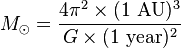# The mass of the Sun

1/ when serching for mesurement Earth-Sun distance, I got this
in the sechon answer, to use this method, we need mass of the Sun but when I searched for Sun' mass http://en.wikipedia.org/wiki/Solar_mass

2/r=GMT^2/(4(πr)^2)

## The Attempt at a Solution

there are the other ways to caculate Sun mass without using AU

## Answers and Replies

What is your question exactly?

The other ways used to caculate the mass of the sun do not use AU.
My homework is how to determine to distance to Sun from Earth, using gavitionval law is one of those ways to measure it. However, using gravitional law requires Sun's Mass and I don't know how to caculate the mass of the Sun without using AU.

What is the course you are studying? What have you studied so far?

Now I'm first year in university so we learn physics as compulsory subject though the main subject is engineering. It was only two month but in hight school, I learned Newton's gravitional law but with some contant like mass of the sun and astronomical unit we weren't taught how to determine/caculate it although we knew its value.

Last edited:
What had you you been studying when you get the homework assigned to you?

That is a lot of material. I am not really sure what you are supposed to use as a given.

On the very first pages you are given the ratio of solar to lunar distance from the Earth. You could use that one. Then you will need to find the lunar distance. It could be derived from the gravity exerted on the Moon by the Earth.